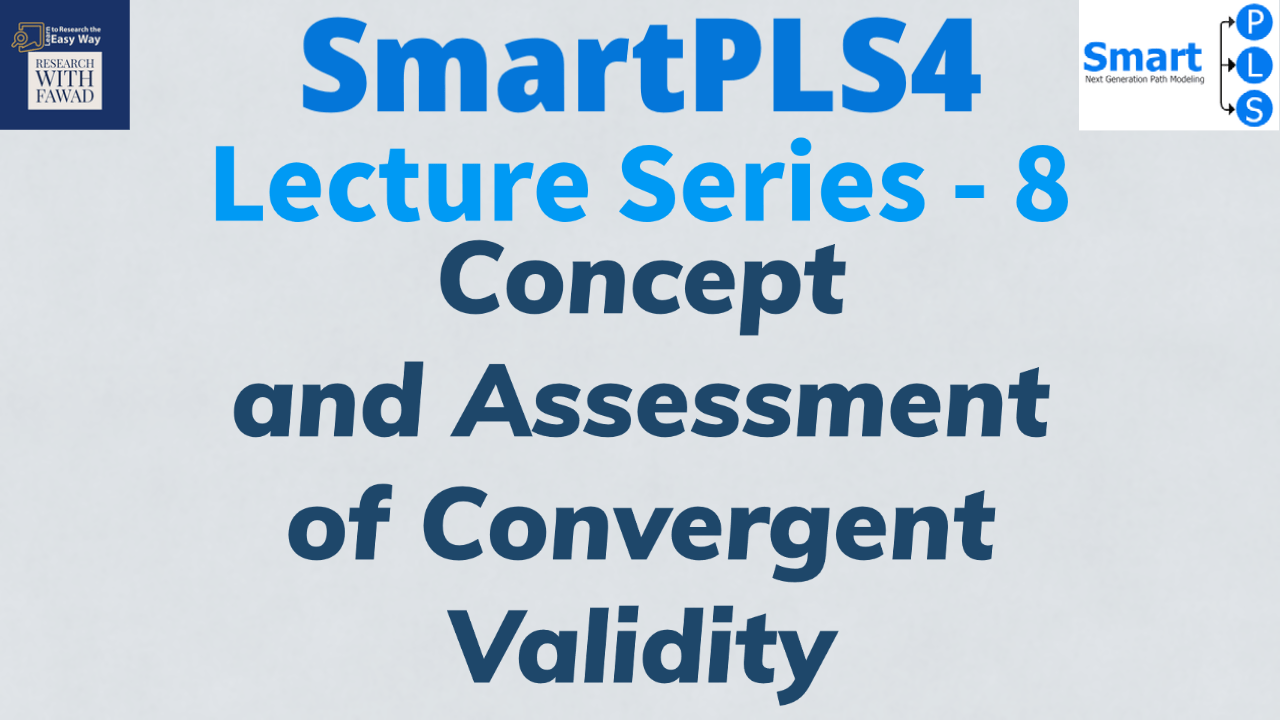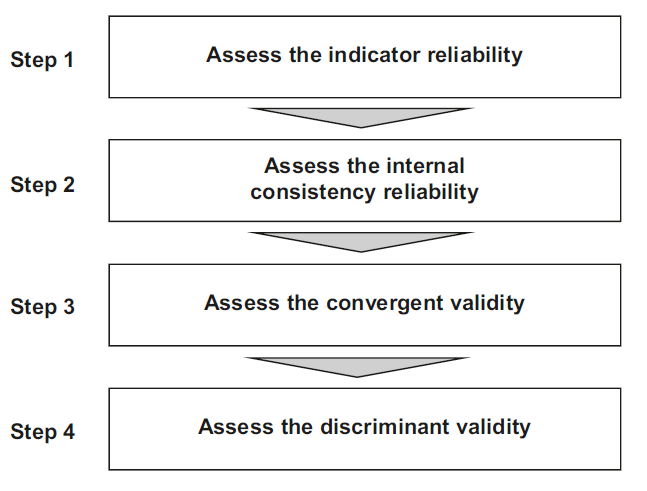# How to Assess Convergent Validity?## Understanding Convergent Validity.

This session discusses in detail the concept and assessment of Convergent Validity using SmartPLS 4. The statistic is assessed as part of the measurement model.

Most often, the constructs are reflective at lower level. Hence, all the lower order constructs in the study are assessed for reliability and validity. The following figure highlights the steps in measurement model assessment.In the last session we discussed how to assess Step 1 and Step 2. In this tutorial, the focus is on convergent validity (Step 3).### What is Convergent Validity?

• The third step is to assess (the) convergent validity of each construct. Convergent validity is the extent to which the construct converges in order to explain the variance of its indicators.
• The metric used for evaluating a construct’s convergent validity is the Average Variance Extracted (AVE) for all indicators on each construct.
• The AVE is defined as the grand mean value of the squared loadings of the indicators associated with the construct (i.e., the sum of the squared loadings divided by the number of indicators).
• Therefore, the AVE is equivalent to the communality of a construct.
• The minimum acceptable AVE is 0.50 – an AVE of 0.50 or higher indicates the construct explains 50 percent or more of the indicators’ variance that make up the construct. Hence, Convergent Validity is Established.

### Reference

Hair Jr, J. F., Hult, G. T. M., Ringle, C. M., Sarstedt, M., Danks, N. P., & Ray, S. (2021). Partial least squares structural equation modeling (PLS-SEM) using R: A workbook.